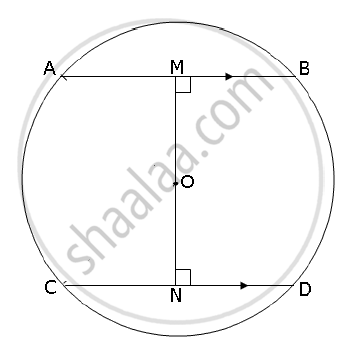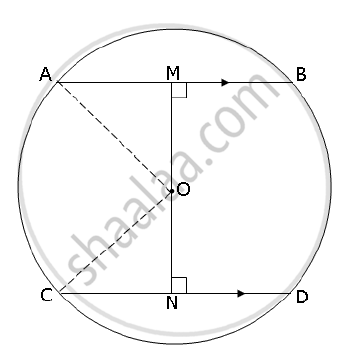Share

In the Figure Given Below Ab and Cd Are Two Parallel Chords and O is the Centre. If the Radius of the Circle is 15 Cm, Find the Distance Mn Between the Two Chords of Length 24 Cm and 18 Cm Respectively. - ICSE Class 10 - Mathematics

ConceptChord Properties - Chords Equidistant from the Center Are Equal (Without Proof)

Question

In the figure given below AB and CD are two parallel chords and O is the centre. If the radius of the circle is 15 cm, find the distance MN between the two chords of length 24 cm and 18 cm respectively.Solution

Construction: Join OA and OBAs OM ⊥ AB and ON ⊥ CD

∴ AM = MB = 24/2 cm = 12 cm

CN = ND = 18/2 cm = 9 cm

:. OM = sqrt(OA^2 -AM^2) = sqrt(15^2 - 12^2) = 9 cm

ON = sqrt(OC^2 - CN^2) = sqrt(15^2 - 9^2 ) = 12 cm

∴ MN = OM + ON = 9 + 12 = 21 cm

Is there an error in this question or solution?

APPEARS IN

2009-2010 (March) (with solutions)
Question 4.2 | 3.00 marks

Video TutorialsVIEW ALL 

Solution In the Figure Given Below Ab and Cd Are Two Parallel Chords and O is the Centre. If the Radius of the Circle is 15 Cm, Find the Distance Mn Between the Two Chords of Length 24 Cm and 18 Cm Respectively. Concept: Chord Properties - Chords Equidistant from the Center Are Equal (Without Proof).
S